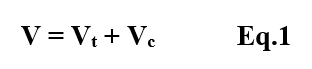### Register Now

The trapezoidal footing formula is used to determine the volume of a trapezoidal footing from the respective drawing specifications. Here, the trapezoidal footing formula is explained and clarified with the help of an example.

## Trapezoidal Footing Formula

As shown in figure-1 below, the trapezoidal footing is a combination two components:

1. Truncated Pyramid
2. Rectangular CuboidFig.1. Trapezoidal Footing- Combination of the Rectangular cuboid and Truncated Pyramid

So,

The volume of trapezoidal footing = Sum of (Volume of truncated pyramid + Volume of  Rectangular Cuboid)

###Fig.2. Plan and Elevation of Trapezoidal Footing

1. The volume of the Truncated Pyramid is given by,From figure-2,

ht = height of the pyramid

A1 =  Area of the bottom surface of the pyramid

A2 = Area of the top surface of the pyramid

A1 = A x B

A2 = a x b

2. The volume of a cuboid is given by,From figure-2,

A = Length of the cuboid

B = Breadth of the cuboid

hc = Height of the cuboid

## Example – Calculation Using Trapezoidal Footing Formula

The trapezoidal footing formula can be explained with an example. Consider a trapezoidal footing arrangement as shown in figure-3 below.Fig.2. Example of Plan and Elevation of Trapezoidal Footing

Here, a square trapezoidal footing is taken into consideration. Let a= 0.8 m and b = 0.8m.

From, the figure-2, A = 1.5m and B = 1.5m

Hence,

1. A1= A x B = 1.5 x 1.5 = 2.25m2
2. A2 = a x b = 0.8 x 0.8m = .64m2
3. ht = 0.3m

Therefore, from Eq.2 and substituting the respective values,

Vt = (0.3/2)( 2.25 + 0.64 + square root of ( 2.25 x 0.64))

= 0.15(2.89 + 1.2)

= 0.614m3

From Eq.3 and substituting the respective values

Vc = 1.5 x 1.5 x 0.2

= 0.45m3

From Eq.1,

Volume of Trapezoidal Footing V = Vt + Vc = 0.614 + 0.45 = 1.064m3

The formula can be used for trapezoidal footing which is either rectangle or square in dimension.

### About Neenu ArjunNeenu is a Civil and Structural Engineer and has experience in Design of Concrete and Steel Structures. She is an Author, Editor and Partner at theconstructor.org.